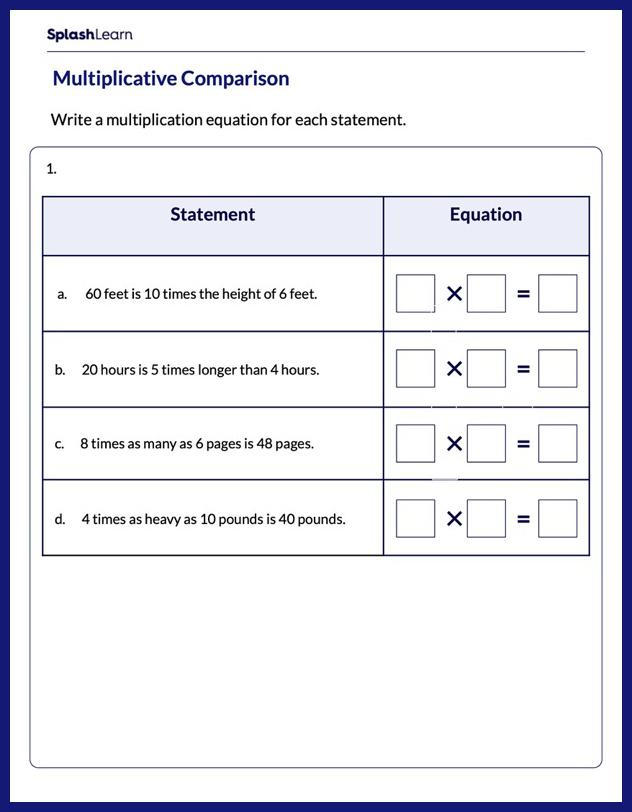# Write the Equation for the Multiplicative Comparison Worksheet

Home > Write the Equation for the Multiplicative ComparisonHelp your child become an expert in multiplication with this worksheet. The worksheet encourages your young mathematician to use their understanding of multiplication to write an equation for the multiplicative comparison situation.